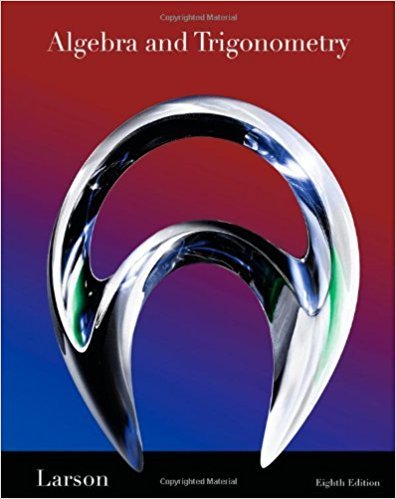×
×

# Solutions for Chapter 7: Analytic Trigonometry## Full solutions for Algebra and Trigonometry | 8th Edition

ISBN: 9781439048474Solutions for Chapter 7: Analytic Trigonometry

Solutions for Chapter 7
4 5 0 414 Reviews
20
2
##### ISBN: 9781439048474

This textbook survival guide was created for the textbook: Algebra and Trigonometry, edition: 8. Chapter 7: Analytic Trigonometry includes 126 full step-by-step solutions. Algebra and Trigonometry was written by and is associated to the ISBN: 9781439048474. Since 126 problems in chapter 7: Analytic Trigonometry have been answered, more than 51590 students have viewed full step-by-step solutions from this chapter. This expansive textbook survival guide covers the following chapters and their solutions.

Key Math Terms and definitions covered in this textbook
• Augmented matrix [A b].

Ax = b is solvable when b is in the column space of A; then [A b] has the same rank as A. Elimination on [A b] keeps equations correct.

• Big formula for n by n determinants.

Det(A) is a sum of n! terms. For each term: Multiply one entry from each row and column of A: rows in order 1, ... , nand column order given by a permutation P. Each of the n! P 's has a + or - sign.

• Cyclic shift

S. Permutation with S21 = 1, S32 = 1, ... , finally SIn = 1. Its eigenvalues are the nth roots e2lrik/n of 1; eigenvectors are columns of the Fourier matrix F.

• Dimension of vector space

dim(V) = number of vectors in any basis for V.

• Dot product = Inner product x T y = XI Y 1 + ... + Xn Yn.

Complex dot product is x T Y . Perpendicular vectors have x T y = O. (AB)ij = (row i of A)T(column j of B).

• Factorization

A = L U. If elimination takes A to U without row exchanges, then the lower triangular L with multipliers eij (and eii = 1) brings U back to A.

• Fast Fourier Transform (FFT).

A factorization of the Fourier matrix Fn into e = log2 n matrices Si times a permutation. Each Si needs only nl2 multiplications, so Fnx and Fn-1c can be computed with ne/2 multiplications. Revolutionary.

• Four Fundamental Subspaces C (A), N (A), C (AT), N (AT).

Use AT for complex A.

• Identity matrix I (or In).

Diagonal entries = 1, off-diagonal entries = 0.

• Multiplicities AM and G M.

The algebraic multiplicity A M of A is the number of times A appears as a root of det(A - AI) = O. The geometric multiplicity GM is the number of independent eigenvectors for A (= dimension of the eigenspace).

• Nullspace matrix N.

The columns of N are the n - r special solutions to As = O.

• Projection p = a(aTblaTa) onto the line through a.

P = aaT laTa has rank l.

• Reduced row echelon form R = rref(A).

Pivots = 1; zeros above and below pivots; the r nonzero rows of R give a basis for the row space of A.

• Reflection matrix (Householder) Q = I -2uuT.

Unit vector u is reflected to Qu = -u. All x intheplanemirroruTx = o have Qx = x. Notice QT = Q-1 = Q.

• Right inverse A+.

If A has full row rank m, then A+ = AT(AAT)-l has AA+ = 1m.

• Solvable system Ax = b.

The right side b is in the column space of A.

• Tridiagonal matrix T: tij = 0 if Ii - j I > 1.

T- 1 has rank 1 above and below diagonal.

• Vector space V.

Set of vectors such that all combinations cv + d w remain within V. Eight required rules are given in Section 3.1 for scalars c, d and vectors v, w.

• Vector v in Rn.

Sequence of n real numbers v = (VI, ... , Vn) = point in Rn.

• Volume of box.

The rows (or the columns) of A generate a box with volume I det(A) I.

×